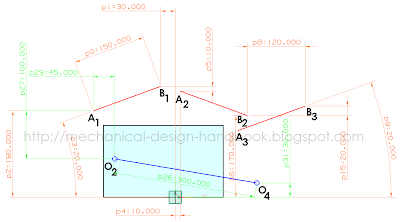## Posts

Showing posts from November, 2010

### Improve math skills of your kids - Learn step-by-step arithmetic from Math games

Math: Unknown - Step-by-step math calculation game for iOS.

Math: Unknown is much more than a math game. It is a step-by-step math calculation game which will teach users how to calculate in the correct order rather than just asking only the final calculated results.

The app consists of four basic arithmetic operations which are addition, subtraction, multiplication and division. In order to get started, users who are new to arithmetic can learn from animated calculation guides showing step-by-step procedures of solving each type of operation. It is also helpful for experienced users as a quick reference.

Generally, addition and subtraction may be difficult for users who just start learning math especially when questions require carrying or borrowing (also called regrouping). The app helps users to visualize the process of carrying and borrowing in the way it will be done on paper. Once users understand how these operations work, they are ready to learn multiplication and division.

For most students, division is considered as the most difficult arithmetic operation to solve. It is a common area of struggle since it requires prior knowledge of both multiplication and subtraction. To help users understand division, the app uses long division to teach all calculation procedures. Relevant multiplication table will be shown beside the question. Users will have to pick a number from the table which go into the dividend. Multiplication of selected number and divisor is automatically calculated, but the users have to do subtraction and drop down the next digit themselves. Learning whole calculation processes will make them master it in no time.

Math: Unknown is a helpful app for students who seriously want to improve arithmetic calculation skills.

### Roberts straight-line mechanismMany engineering applications require things move in a linear fashion or "straight-line motion". We can use a linear motion guide that can guide a device accurately along a straight line. Manufacturing know-how of most linear guide manufacturers has let us keep expanding the range of linear guidance. The picture shown here is an example of commercially available linear guides from THK. This Linear Ball Slide is a lightweight, compact, limited stroke linear guide unit that operates with very low sliding resistance. It excels in high-speed responsive performance due to its very small frictional factor and low inertia.

In the late seventeenth century, before the development of the milling machine, it was extremely difficult to machine straight, flat surfaces. For this reason, good prismatic pairs without backlash were not easy to make. During that era, much thought was given to the problem of attaining a straight-line motion as a part of the coupler curve of a linkage having …

### 3-Position Synthesis with Inversion Method using Unigraphics NX4 Sketch - Part 3

In [3-Position Synthesis with Inversion Method using Unigraphics NX4 Sketch - Part 2], we've shown an example to do three-position synthesis of  a four-bar linkage using inversion method in Unigraphics NX4 sketch.

We can make a quick motion simulation using "animate dimension" command in Unigraphics (UG) NX4 sketch. Just draw lines as per a sketch and add one driving dimension as shown below. Then use animate dimension command to set the lower and upper limits, for this case they're minimum and maximum angles.

### 3-Position Synthesis with Inversion Method using Unigraphics NX4 Sketch - Part 2In previous posts, the fixed pivot points were determined from the moving pivot points. We can get result that can't be fitted in our design due to space limit. The principle of inversion can be applied to solve this problem. The first step is to find the three positions of the ground plan that correspond to the three desired coupler positions.